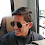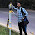# How to Check Bearing Capacity of Soil at Site?

Bearing capacity is the capacity of soil to support or withstand the load applied to the ground.

Without soil, construction of civil engineering structures like: buildings, dams, bridges, etc. is not possible. All the loads applied to the structures gets distributed among the soil through the foundation of the structure.

Bearing capacity of soil depends upon various factors like: types of soil, it’s shear strength, permeability, density, etc. Also, the deeper the foundation of the structure the higher the bearing capacity of the soil.

Procedures to determine the bearing capacity of soil at site are:

Step 1: At first, we excavate a small pit of certain depth at the ground               whose bearing capacity is to be determined.

We should determine the bearing capacity of soil in certain depth than in the surface because we don’t place footing on the surface rather in certain depth and also the load is distributed in the soil at certain depth, not on the surface.

Step 2: Now take a ball or cube of certain size whose dimensions and               weight are known.

Step 3: Drop the ball or cube with free fall in the excavated pit from               certain height for several times.

Step 4: Now, measure the average depth of the impression on the                       excavated pit. From figure, 'd' is the depth of impression.

Step 5: Finally, take the average value of the depth of the impression.

Now, the formula to determine the bearing capacity is:

Bearing Capacity= R = (w*h)/d

Where, R= ultimate resistance of soil (in kg)

d= average depth of impression (in cm)

w= weight of solid ball or cube (in kg)

h= height of fall (in cm)

Example: Take a Cube of known dimension and weight

Weight of cube= 1kg

Dimension of cube= 15cm * 15cm * 15cm

Height of fall= 200cm

Depth of impression: 1.5cm, 2cm, 2cm, 1cm

Average depth of impression= (1.5+2+2+1)/4 = 1.625cm

Hence, resistance of soil (R) =  (w*h)/d

= (1*200)/1.625

=123.076 kg

Then, Resistance of soil per unit area=Resistance of Soil(R) / Contact                                                                                             area of cube(A)

= 0.547 kg/cm^2

Hence, 1cm^2 area of soil can bear a load up to 1.547 kg.

We don’t take this reading as applicable resistance of soil in site because we have to take safety precautions and this above resistance is the maximum capacity of soil to withstand the load. If we built a structure taking consideration as resistance of soil per unit area as above then, slightly increase in load might causes failure of structure.

Hence, for safety protocol and to assure the safety of our structure we take safe bearing capacity of soil as the bearing capacity of soil while constructing any structure.

Hence,

Safe bearing Capacity of soil= R / (A*FOS)

Where, FOS = factor of safety (which is 2 to 3 for a soil)

=123.076 / 15* 15 *2

=0.273 kg/cm^2

Hence, we take half or one-third of the resistance of soil ( or bearing capacity of soil) as a safe bearing capacity of soil.

Different types of soil with their bearing capacity

 Types of Soil Safe Bearing Capacity Fine Sand 4.40 kg/cm^2 Medium Sand 2.45 kg/cm^2 Very Soft Clay 0.52 kg/cm^2 Rock 33.10 kg/cm^2 Soft Rock 4.49 kg/cm^2 Coarse Sand 4.40 kg/cm^2 Stiff Clay 1 kg/cm^2

Important Concept:

We know the formula to determine the bearing capacity of soil is: R=(w*h) / d.Hence, if we use same object and throw the object from same distance for several times, then ‘W’ and ‘h’ remains constant making equation R. This means higher the depth of impression lowers the bearing capacity of soil and lower the depth of impression higher the bearing capacity of soil. Hence, for higher bearing capacity of soil, the observed depth of impression should be lower.

1.informative xa mohan sir...

1.2.Very well explained mate.

1.I am glad you liked it.

3.Thanks for the information bro😊❤.

1.My pleasure.

4.The Swedish software operator presents on-line casinos the most clutter-breaking gambling instruments for the sports betting ecosystem. Due to the privacy provided by on-line gambling, you’ll still discover many on-line casinos Korean gamers can gamble 코인카지노 at right now, including many main brands from the business that operate on a world scale. Nearly all of those casinos are set up offshore, which technically doesn’t make them unlawful.

5.This comment has been removed by the author.

6.Mobile crushing refers to the process of reducing large rocks into smaller-sized materials using a mobile crusher.
Limestone Quarry